# Chemical Equations Counting atoms Balancing chemical equations Classifying

• Slides: 25Chemical Equations üCounting atoms üBalancing chemical equations üClassifying chemical reactions üWriting word & formula equations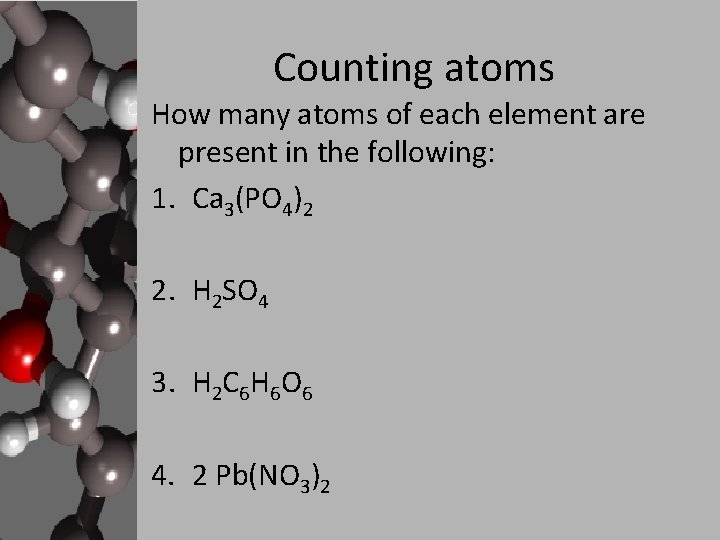Counting atoms How many atoms of each element are present in the following: 1. Ca 3(PO 4)2 2. H 2 SO 4 3. H 2 C 6 H 6 O 6 4. 2 Pb(NO 3)2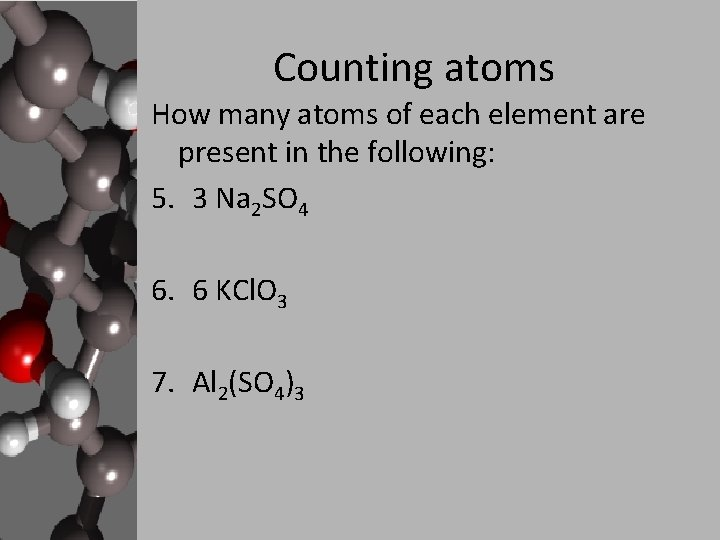Counting atoms How many atoms of each element are present in the following: 5. 3 Na 2 SO 4 6. 6 KCl. O 3 7. Al 2(SO 4)3Counting atoms In the following equations, one of the reactants is in bold type. Do you find the same number of atoms of this element on both sides of the equation? 8. 2 KCl + Pb(NO 3)2 KNO 3 + Pb. Cl 2 9. (NH 4)3 PO 4 + Sr(OH)2 Sr 3(PO 4)2 + NH 4 OH 10. 6 CO 2 + 6 H 2 O C 6 H 12 O 6 + 6 O 2 11. 4 Si 2 H 3 + 11 O 2 4 Si. O 2 + 6 H 2 O 12. Fe 2(C 2 O 4)3 3 Fe. C 2 O 4 + 2 CO 2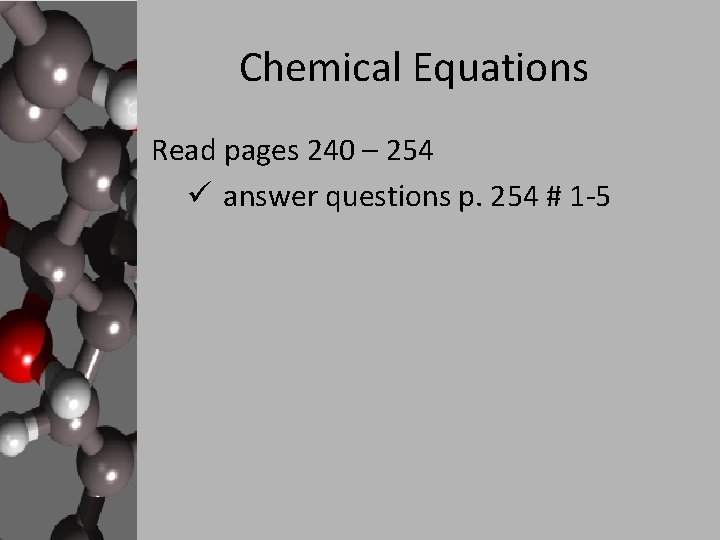Chemical Equations Read pages 240 – 254 ü answer questions p. 254 # 1 -5Balancing Chemical Equations According to the law of conservation of mass, matter cannot be created or destroyed. So… in a chemical reaction atoms cannot be created or destroyed – if there are 4 atoms of nitrogen on the reactants side of an equation, there must be 4 atoms of nitrogen on the products side. Pb(NO 3)4 + Ca. SO 4 ---> Pb(SO 4)2 + Ca(NO 3)2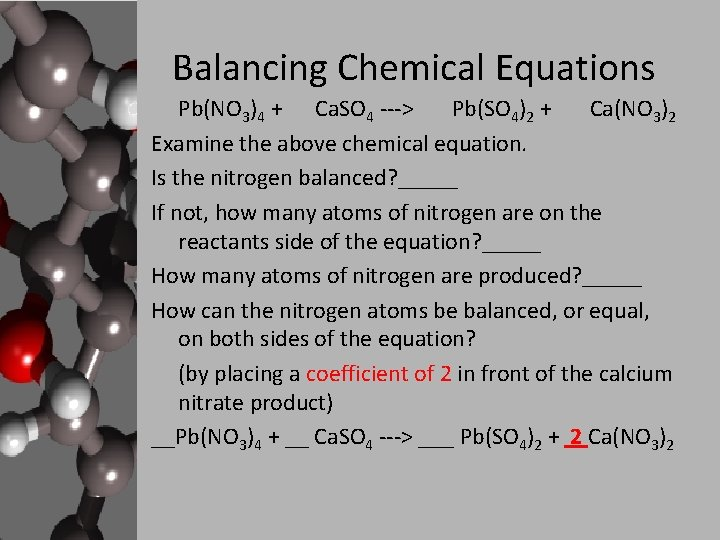Balancing Chemical Equations Pb(NO 3)4 + Ca. SO 4 ---> Pb(SO 4)2 + Ca(NO 3)2 Examine the above chemical equation. Is the nitrogen balanced? _____ If not, how many atoms of nitrogen are on the reactants side of the equation? _____ How many atoms of nitrogen are produced? _____ How can the nitrogen atoms be balanced, or equal, on both sides of the equation? (by placing a coefficient of 2 in front of the calcium nitrate product) __Pb(NO 3)4 + __ Ca. SO 4 ---> ___ Pb(SO 4)2 + 2 Ca(NO 3)2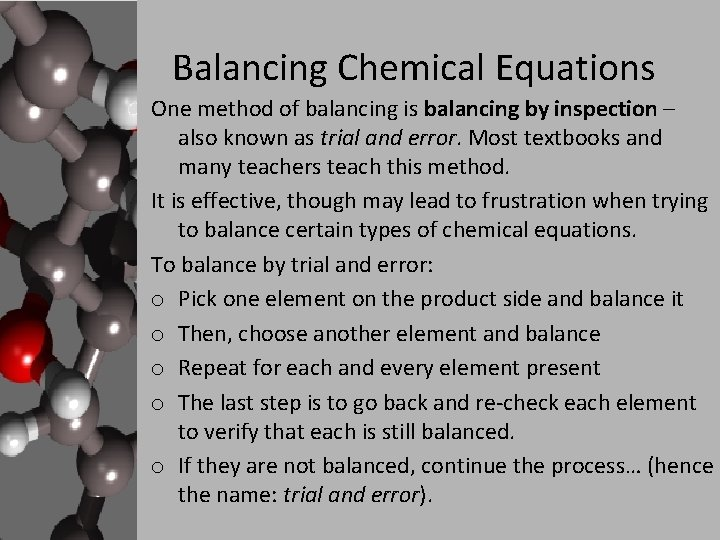Balancing Chemical Equations One method of balancing is balancing by inspection – also known as trial and error. Most textbooks and many teachers teach this method. It is effective, though may lead to frustration when trying to balance certain types of chemical equations. To balance by trial and error: o Pick one element on the product side and balance it o Then, choose another element and balance o Repeat for each and every element present o The last step is to go back and re-check each element to verify that each is still balanced. o If they are not balanced, continue the process… (hence the name: trial and error).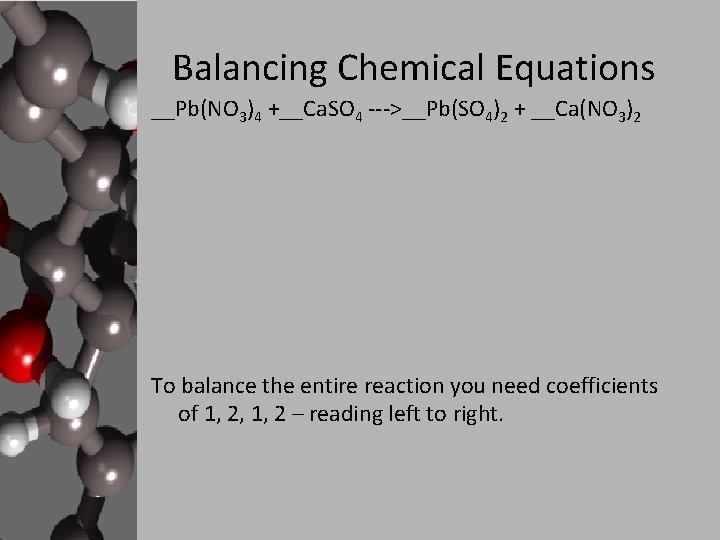Balancing Chemical Equations __Pb(NO 3)4 +__Ca. SO 4 --->__Pb(SO 4)2 + __Ca(NO 3)2 To balance the entire reaction you need coefficients of 1, 2, 1, 2 – reading left to right.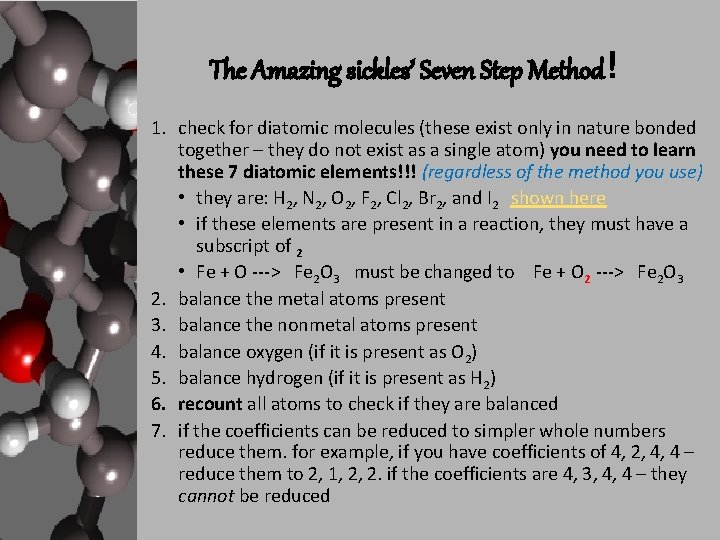The Amazing sickles’ Seven Step Method! 1. check for diatomic molecules (these exist only in nature bonded together – they do not exist as a single atom) you need to learn these 7 diatomic elements!!! (regardless of the method you use) • they are: H 2, N 2, O 2, F 2, Cl 2, Br 2, and I 2 shown here • if these elements are present in a reaction, they must have a subscript of 2 • Fe + O ---> Fe 2 O 3 must be changed to Fe + O 2 ---> Fe 2 O 3 2. balance the metal atoms present 3. balance the nonmetal atoms present 4. balance oxygen (if it is present as O 2) 5. balance hydrogen (if it is present as H 2) 6. recount all atoms to check if they are balanced 7. if the coefficients can be reduced to simpler whole numbers reduce them. for example, if you have coefficients of 4, 2, 4, 4 – reduce them to 2, 1, 2, 2. if the coefficients are 4, 3, 4, 4 – they cannot be reduced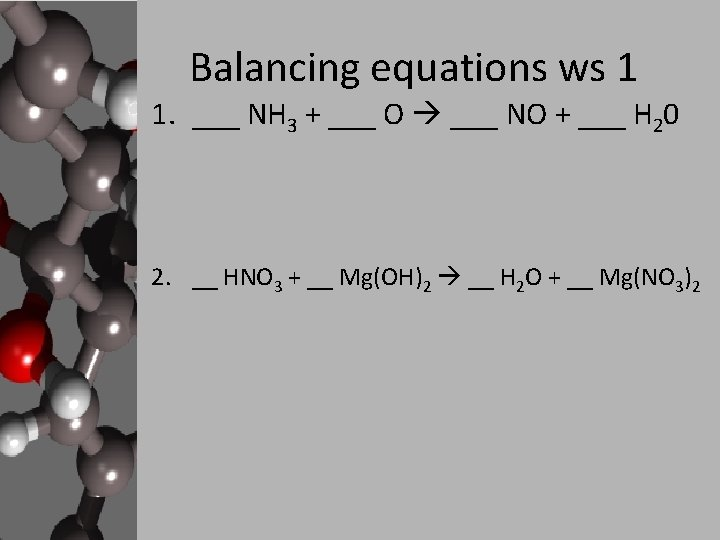Balancing equations ws 1 1. ___ NH 3 + ___ O ___ NO + ___ H 20 2. __ HNO 3 + __ Mg(OH)2 __ H 2 O + __ Mg(NO 3)2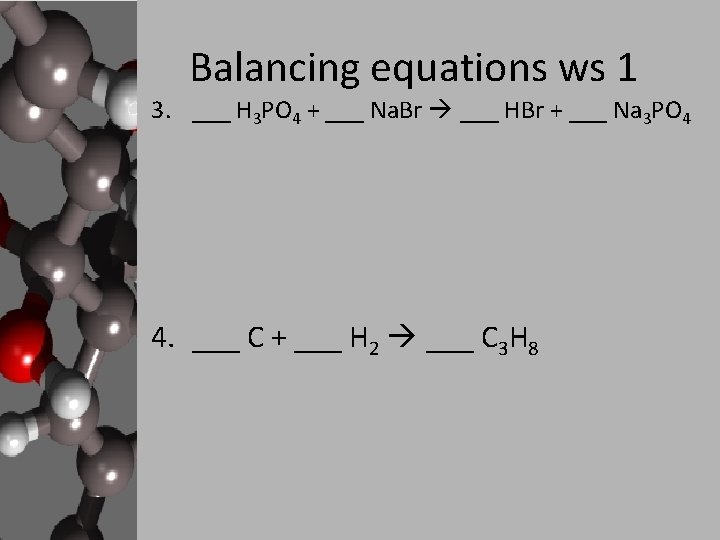Balancing equations ws 1 3. ___ H 3 PO 4 + ___ Na. Br ___ HBr + ___ Na 3 PO 4 4. ___ C + ___ H 2 ___ C 3 H 8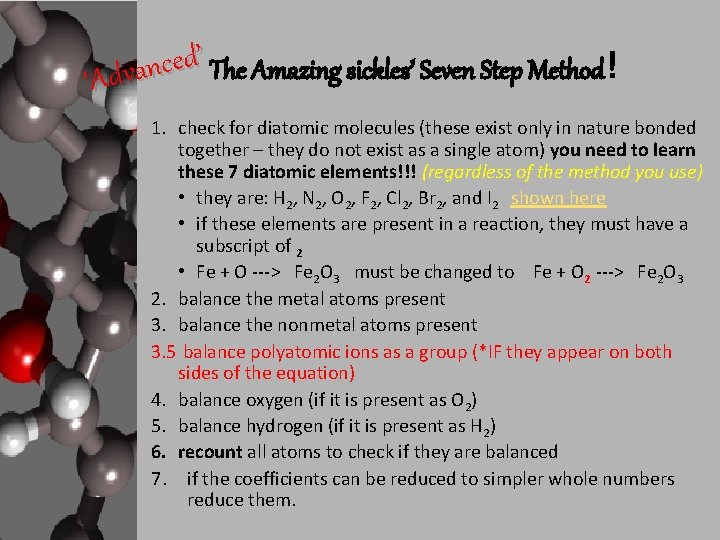’ The Amazing sickles’ Seven Step Method! d e c n a ‘Adv 1. check for diatomic molecules (these exist only in nature bonded together – they do not exist as a single atom) you need to learn these 7 diatomic elements!!! (regardless of the method you use) • they are: H 2, N 2, O 2, F 2, Cl 2, Br 2, and I 2 shown here • if these elements are present in a reaction, they must have a subscript of 2 • Fe + O ---> Fe 2 O 3 must be changed to Fe + O 2 ---> Fe 2 O 3 2. balance the metal atoms present 3. balance the nonmetal atoms present 3. 5 balance polyatomic ions as a group (*IF they appear on both sides of the equation) 4. balance oxygen (if it is present as O 2) 5. balance hydrogen (if it is present as H 2) 6. recount all atoms to check if they are balanced 7. if the coefficients can be reduced to simpler whole numbers reduce them.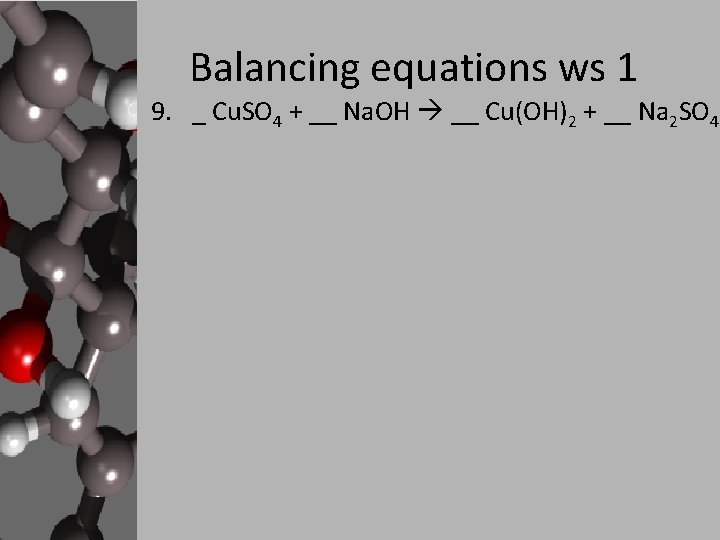Balancing equations ws 1 9. _ Cu. SO 4 + __ Na. OH __ Cu(OH)2 + __ Na 2 SO 4Classifying Chemical Reactions Read pages 256 – 263 ü answer questions p. 264 # 1 -4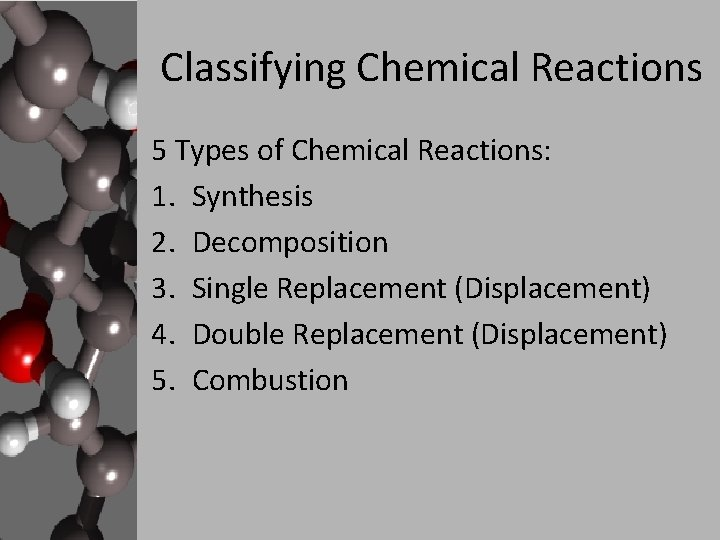Classifying Chemical Reactions 5 Types of Chemical Reactions: 1. Synthesis 2. Decomposition 3. Single Replacement (Displacement) 4. Double Replacement (Displacement) 5. Combustion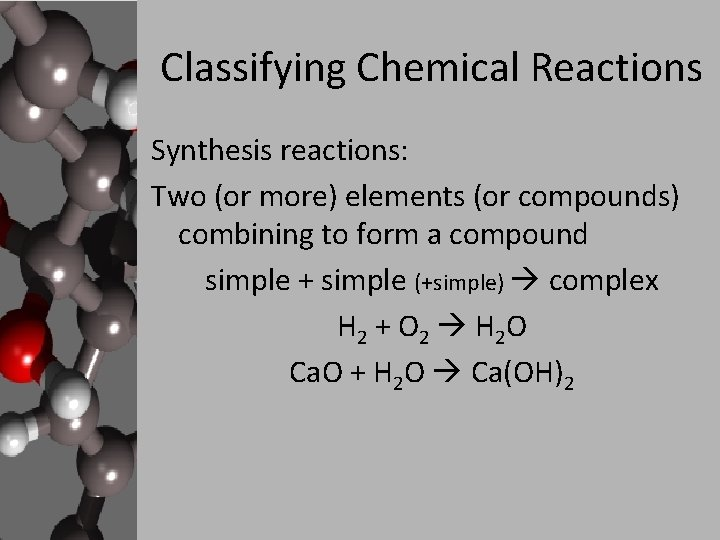Classifying Chemical Reactions Synthesis reactions: Two (or more) elements (or compounds) combining to form a compound simple + simple (+simple) complex H 2 + O 2 H 2 O Ca. O + H 2 O Ca(OH)2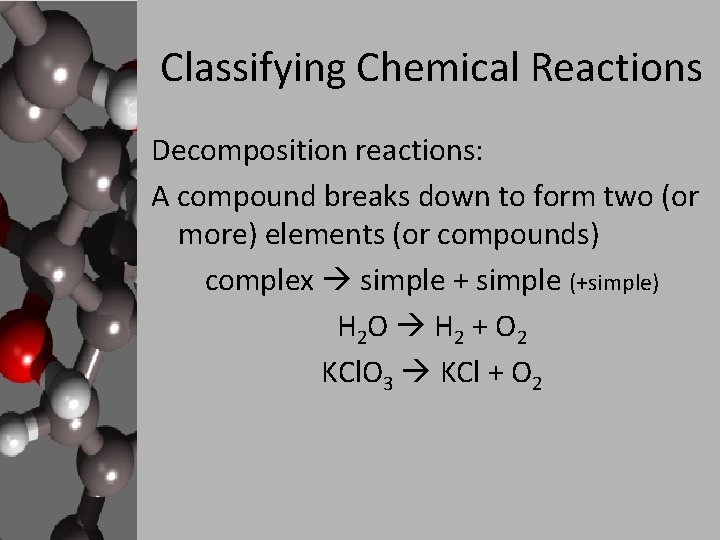Classifying Chemical Reactions Decomposition reactions: A compound breaks down to form two (or more) elements (or compounds) complex simple + simple (+simple) H 2 O H 2 + O 2 KCl. O 3 KCl + O 2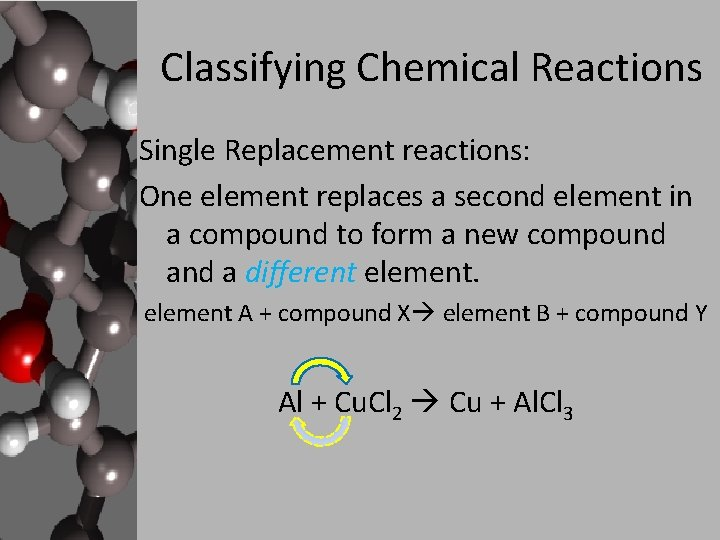Classifying Chemical Reactions Single Replacement reactions: One element replaces a second element in a compound to form a new compound a different element A + compound X element B + compound Y Al + Cu. Cl 2 Cu + Al. Cl 3Classifying Chemical Reactions Double Replacement reactions: One element in a compound replaces an element in another compound (& the 2 nd element takes the 1 st element’s place). compound A + compound B compound X + compound Y Cu(NO 3)2 + Na. OH Cu(OH)2 + Na. NO 3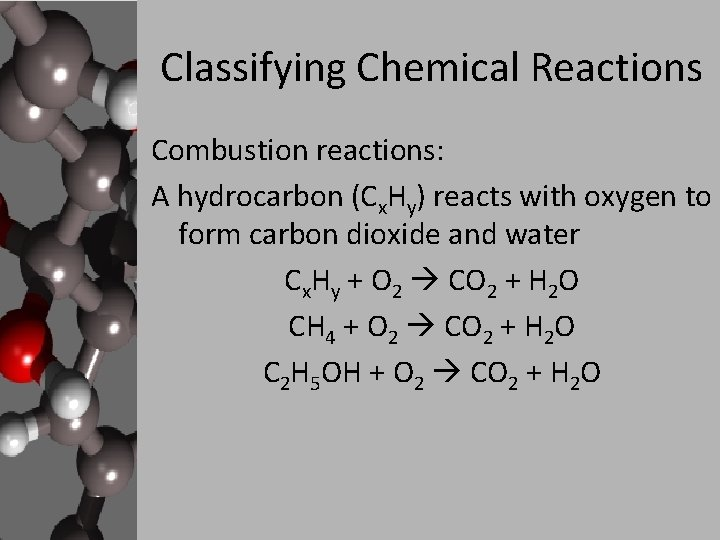Classifying Chemical Reactions Combustion reactions: A hydrocarbon (Cx. Hy) reacts with oxygen to form carbon dioxide and water Cx. Hy + O 2 CO 2 + H 2 O CH 4 + O 2 CO 2 + H 2 O C 2 H 5 OH + O 2 CO 2 + H 2 O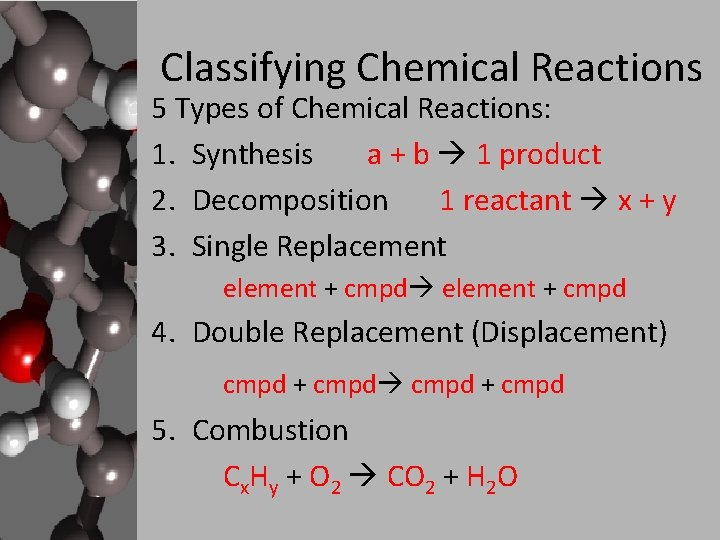Classifying Chemical Reactions 5 Types of Chemical Reactions: 1. Synthesis a + b 1 product 2. Decomposition 1 reactant x + y 3. Single Replacement element + cmpd 4. Double Replacement (Displacement) cmpd + cmpd 5. Combustion Cx. Hy + O 2 CO 2 + H 2 OClassifying Chemical Reactions 1. 2. 3. 4. 5. 6. 7. Hg. O Hg + O D Na + H 2 O Na. OH + H 2 SR S 8 + O 2 SO 3 S HNO 3 + Mg(OH)2 H 2 O + Mg(NO 3)2 DR CF 4 + Br CBr 4 + F SR C 7 H 16 + O 2 CO 2 + H 2 O C Si 2 H 3 + O 2 Si. O 2 + H 2 O DR’ r e p u ‘S The Amazing sickles’ Combustion Method! 1. double the hydrocarbon 2. balance the C 3. balance the H 4. balance the O (using 02…) 5. reduce, if necessary’ r e p u ‘S The Amazing sickles’ Combustion Method! ___C 7 H 16 + ___O 2 ___CO 2 + ___H 2 O ___ C 3 H 7 OH + ___ O 2 ___ CO 2 + ___ H 2 O